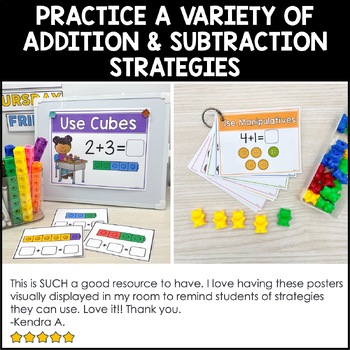# Math Strategies Posters (Addition Strategies and Subtraction Strategies)Subject
Resource Type
File Type

PDF

(27 MB|35 pages)
Product Rating
4.0
(68 Ratings)
Standards
• Product Description
• StandardsNEW

Teaching your students how to add and subtract can be really, really hard! I am always trying to find concrete ways to help the concepts stick, and one strategy never works for every single student in my class. We use many, many strategies throughout the year and I found myself always drawing the new strategy up on the board during our mini-lesson. I finally turned those drawings into posters!

These math strategy posters are perfect to use as a reference in your classroom as your students are learning addition and subtraction. Hang the posters on a bulletin board, use them during a mini-lesson, or print multiple to a page to use as a personal reference guide for your students. 30 posters are included, both for addition and subtraction!

*To reduce the size of the posters, you can change your printer settings before printing.

What's included?

• Count on (2 posters)
• Combine & count
• Use a ten frame
• Draw a picture
• Use a number line
• Use mental math
• Make 10
• Find doubles
• Use manipulatives
• Use tally marks
• Draw dots
• Use counters
• Use cubes
• Write an equation
• Flip flop facts
• Doubles +1

12 subtraction strategies

• Count back
• Cross out
• Use a ten frame
• Draw a picture
• Use a number line
• Use mental math
• Use manipulatives
• Draw dots
• Use counters
• Use cubes
• Write an equation

Need math strategy posters in Spanish? Check them out here.

You can find more math resources in my store below:

Fluently add and subtract within 5.
For any number from 1 to 9, find the number that makes 10 when added to the given number, e.g., by using objects or drawings, and record the answer with a drawing or equation.
Decompose numbers less than or equal to 10 into pairs in more than one way, e.g., by using objects or drawings, and record each decomposition by a drawing or equation (e.g., 5 = 2 + 3 and 5 = 4 + 1).
Solve addition and subtraction word problems, and add and subtract within 10, e.g., by using objects or drawings to represent the problem.
Represent addition and subtraction with objects, fingers, mental images, drawings, sounds (e.g., claps), acting out situations, verbal explanations, expressions, or equations.
Total Pages
35 pages
N/A
Teaching Duration
N/A
Report this Resource to TpT
Reported resources will be reviewed by our team. Report this resource to let us know if this resource violates TpT’s content guidelines.